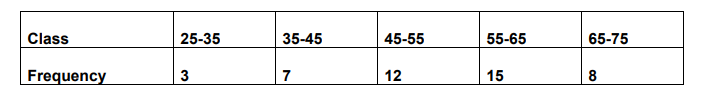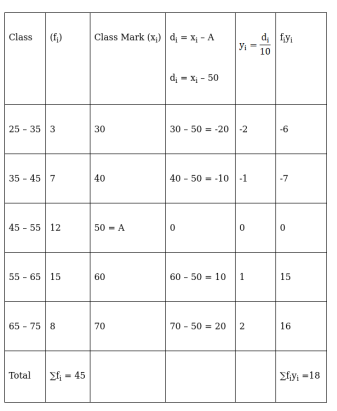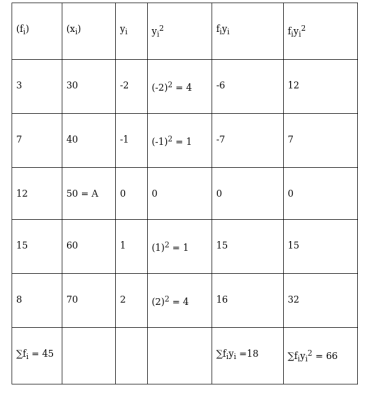# Using short cut method, find the mean, variation and standard deviation for the data :

Question:

Using short cut method, find the mean, variation and standard deviation for the data :Solution:

Here, we apply the step deviation method with A = 50 and h = 10

To find: MEANNow, $\operatorname{Mean}(\overline{\mathrm{x}})=\mathrm{a}+\mathrm{h}\left(\frac{\sum \mathrm{f}_{\mathrm{i}} \mathrm{y}_{\mathrm{i}}}{\sum \mathrm{f}_{\mathrm{i}}}\right)$

$\Rightarrow \overline{\mathrm{x}}=50+10\left(\frac{18}{45}\right)$

$\Rightarrow \overline{\mathrm{x}}=50+\frac{2 \times 18}{9}$

$\Rightarrow \overline{\mathrm{x}}=50+4$

$\Rightarrow \overline{\mathrm{x}}=54$

To find: VARIANCEVariance, $\sigma^{2}=\frac{\mathrm{h}^{2}}{\mathrm{~N}^{2}}\left[\mathrm{~N} \sum \mathrm{f}_{\mathrm{i}} \mathrm{y}_{\mathrm{i}}^{2}-\left(\sum \mathrm{f}_{\mathrm{i}} \mathrm{y}_{\mathrm{i}}\right)^{2}\right]$

$=\frac{(10)^{2}}{(45)^{2}}\left[45 \times 66-(18)^{2}\right]$

$=\frac{10 \times 10}{45 \times 45}[2970-324]$

$=\frac{4}{81}$

$=130.67$

To find: STANDARD DEVIATION

Standard Deviation $(\sigma)=\sqrt{\text { Variance }}$

$=\sqrt{130.67}$

$=11.43$